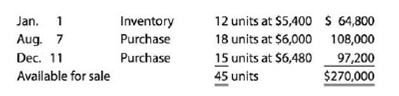# Periodic inventory using FIFO, LIFO, and weighted average cost methods The units of an item available for sale during the year were as follows: There are 14 units of the item in the physical inventory at December 31. The periodic inventory system is used. Determine the inventory cost using (a) the first-in, first-out (FIFO) method; (b) the last-in, first-out (LIFO) method; and (c) the weighted average cost method.### Accounting

27th Edition
WARREN + 5 others
Publisher: Cengage Learning,
ISBN: 9781337272094### Accounting

27th Edition
WARREN + 5 others
Publisher: Cengage Learning,
ISBN: 9781337272094

#### Solutions

Chapter
Section
Chapter 7, Problem 7.5APE
Textbook Problem

## Periodic inventory using FIFO, LIFO, and weighted average cost methodsThe units of an item available for sale during the year were as follows:There are 14 units of the item in the physical inventory at December 31. The periodic inventory system is used. Determine the inventory cost using (a) the first-in, first-out (FIFO) method; (b) the last-in, first-out (LIFO) method; and (c) the weighted average cost method.

Expert Solution

(a)

To determine

Periodic inventory system is a system in which the inventory is updated in the accounting records on a periodic basis such as at the end of each month, quarter or year. In other words, it is an accounting method which is used to determine the amount of inventory at the end of each accounting period.

First-in-First-Out:

In First-in-First-Out method, the costs of the initially purchased items are considered as cost of goods sold, for the items which are sold first. The value of the ending inventory consists of the recent purchased items.

Last-in-Last-Out:

In Last-in-First-Out method, the costs of last purchased items are considered as the cost of goods sold, for the items which are sold first. The value of the closing stock consists of the initial purchased items.

Weighted -average cost method:

Under weighted average cost method, the company calculates a new average cost after every purchase is made. It is determined by dividing the cost of goods available for sale by the units on hand.

To determine: The ending inventory using the assumed cash flow methods of FIFO method.

### Explanation of Solution

Calculate the ending inventory using FIFO method.

 Ending Inventory Date Units Unit Cost
Expert Solution

(b)

To determine
The ending inventory using the assumed cash flow methods of LIFO method.

Expert Solution

(c)

To determine
The ending inventory using the assumed cash flow methods of average cost method.

### Want to see the full answer?

Check out a sample textbook solution.See solution

### Want to see this answer and more?

Bartleby provides explanations to thousands of textbook problems written by our experts, many with advanced degrees!

See solution

Find more solutions based on key concepts
Show solutions
What is participative budgeting? Discuss some of its advantages.

Managerial Accounting: The Cornerstone of Business Decision-Making

Use a production possibilities frontier to describe the idea of efficiency.

Principles of Macroeconomics (MindTap Course List)

Does interest rate parity imply that interest rates are the same in all countries?

Fundamentals of Financial Management (MindTap Course List)

TIME VALUE OF MONEY Answer the following questions: a. Assuming a rate of 10% annually, find the FV of 1,000 af...

Fundamentals of Financial Management, Concise Edition (with Thomson ONE - Business School Edition, 1 term (6 months) Printed Access Card) (MindTap Course List)